Skip to main content

# 4.3 Stability of Cycloalkanes - Ring Strain

Objectives

After completing this section, you should be able to

1. describe the Baeyer strain theory.
2. describe how the measurement of heats of combustion can be used to provide information about the amount of strain present in an alicyclic ring.
3. explain the inadequacies of the Baeyer strain theory.
4. determine which of two similar compounds is likely to be the most stable, by assessing such factors as angle strain, torsional strain and steric strain.

Key Terms

Make certain that you can define, and use in context, the key terms below.

• angle strain
• heat of combustion
• steric strain
• torsional strain

Cycloalkanes have one or more rings of carbon atoms. The simplest examples of this class consist of a single, unsubstituted carbon ring, and these form a homologous series similar to the unbranched alkanes. The IUPAC names of the first five members of this series are given in the following table. The last (yellow shaded) column gives the general formula for a cycloalkane of any size. If a simple unbranched alkane is converted to a cycloalkane two hydrogen atoms, one from each end of the chain, must be lost. Hence the general formula for a cycloalkane composed of n carbons is CnH2n. Although a cycloalkane has two fewer hydrogens than the equivalent alkane, each carbon is bonded to four other atoms so such compounds are still considered to be saturated with hydrogen.

Table 4.3.1: Examples of Simple Cycloalkanes
Name Cyclopropane Cyclobutane Cyclopentane Cyclohexane Cycloheptane Cycloalkane
Molecular
Formula
C3H6 C4H8 C5H10 C6H12 C7H14 CnH2n
Structural
Formula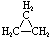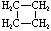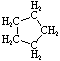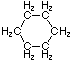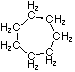(CH2)n
Line
Formula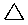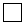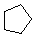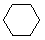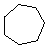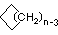## The Baeyer Theory on the Strain in Cycloalkane Rings

Many of the properties of cyclopropane and its derivatives are similar to the properties of alkenes. In 1890, the famous German organic chemist, A. Baeyer, suggested that cyclopropane and cyclobutane derivatives are different from cyclopentane and cyclohexane, because their C—C—C angles cannot have the tetrahedral value of 109.5°. At the same time, Baeyer hypothesized that the difficulties encountered in synthesizing cycloalkane rings from C7 upward was the result of the angle strain that would be expected if the large rings were regular planar polygons (see Table 12-3). Baeyer also believed that cyclohexane had a planar structure like that shown in Figure 12-2, which would mean that the bond angles would have to deviate 10.5° from the tetrahedral value. However, in 1895, the then unknown chemist H. Sachse suggested that cyclohexane exists in the strain-free chair and boat forms discussed in Section 12-3. This suggestion was not accepted at the time because it led to the prediction of several possible isomers for compounds such as chlorocyclohexane (cf. Exercise 12-4). The idea that such isomers might act as a single substance, as the result of rapid equilibration, seemed like a needless complication, and it was not until 1918 that E. Mohr proposed a definitive way to distinguish between the Baeyer and Sachse cyclohexanes. As will be discussed in Section 12-9, the result, now known as the Sachse-Mohr theory, was complete confirmation of the idea of nonplanar large rings.

Table 4.3.2: Strain in Cycloalkane Rings and Heats of Combustion of Cycloalkanes
Compound n Angle Strain at each CH2 Heat of Combustion ΔHo (kcal/mol) Heat of Combustion ΔHo per CH2/N (kcal/mol) Total Strain (kcal/mol)
ethene 2 109.5 337.2 168.6 22.4
cyclopropane 3 49.5 499.9 166.6 27.7
cyclobutane 4 19.5 655.9 164.0 26.3
cyclopentane 5 1.5 793.4 158.7 6.5
cyclohexane 6 10.5 944.8 157.5 0.4
cycloheptane 7 19.1 1108.1 158.4 6.3
cyclooctane 8 25.5 1268.9 158.6 9.7
cyclononane 9 30.5 1429.5 158.8 12.9
cyclodecane 10 34.5 1586.1 158.6 12.1
cyclopentadecane 15 46.5 2362.5 157.5 1.5
open chain alkane 157.4 -

One of the most interesting developments in ‘the stereochemistry of organic compounds in recent years has been the demonstration that transcyclooctene (but not the cis isomer) can be resolved into stable chiral isomers (enantiomers, Section 5-IB). In general, a trans-cycloalkene would not be expected to be resolvable because of the possibility for formation of achiral conformations with a plane of symmetry. Any conformation with all of the carbons in a plane is such an achiral conformation (Figure 12-20a). However, when the chain connecting the ends of the double bond is short, as in trans-cyclooctene, steric hindrance and steric strain prevent easy.

## Ring Strain in CycloalkanesRing Strain occurs because the carbons in cycloalkanes are sp3 hybridized, which means that they do not have the expected ideal bond angle of 109.5o ; this causes an increase in the potential energy because of the desire for the carbons to be at an ideal 109.5o. An example of ring strain can be seen in the diagram of cyclopropane below in which the bond angle is 60o between the carbons.The reason for ring strain can be seen through the tetrahedral carbon model. The C-C-C bond angles in cyclopropane (diagram above) (60o) and cyclobutane (90o) are much different than the ideal bond angle of 109.5o. This bond angle causes cyclopropane and cyclobutane to have a high ring strain. However, molecules, such as cyclohexane and cyclopentane, would have a much lower ring strain because the bond angle between the carbons is much closer to 109.5o.

Below are some examples of cycloalkanes. Ring strain can be seen more prevalently in the cyclopropane and cyclobutane models.Below is a chart of cycloalkanes and their respective heats of combustion ( ΔHcomb). The ΔHcomb value increases as the number of carbons in the cycloalkane increases (higher membered ring), and the ΔHcomb/CH2 ratio decreases. The increase in ΔHcomb can be attributed to the greater amount of London Dispersion forces. However, the decrease in ΔHcomb/CH2can be attributed to a decrease in the ring strain.Certain cycloalkanes, such as cyclohexane, deal with ring strain by forming conformers. A conformer is a stereoisomer in which molecules of the same connectivity and formula exist as different isomers, in this case, to reduce ring strain. The ring strain is reduced in conformers due to the rotations around the sigma bonds. More about cyclohexane and its conformers can be seen here.

### Different Types of StrainThere are many different types of strain that occur with cycloalkanes. In addition to ring strain, there is also transannular strain, eclipsing, or torsional strain and bond angle strain.Transannular strain exists when there is steric repulsion between atoms. Eclipsing (torsional) strain exists when a cycloalkane is unable to adopt a staggered conformation around a C-C bond, and bond angle strain is the energy needed to distort the tetrahedral carbons enough to close the ring. The presence of angle strain in a molecule indicates that there are bond angles in that particular molecule that deviate from the ideal bond angles required (i.e., that molecule has conformers).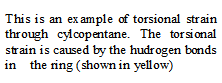## Exercises

#### Questions

Q4.3.1

trans-1,2-Dimethylcyclobutane is more stable than cis-1,2-dimethylcyclobutane. Explain this observation.

#### Solutions

S4.3.1

The trans form does not have eclipsing methyl groups, therefore lowering the energy within the molecule. It does however have hydrogen-methyl interactions, but are not as high in energy than methyl-methyl interactions.